Next: Resonant Depolarization Up: Introduction Previous: Spin Dynamics   Contents

## Depolarizing Effects

In a real storage ring like SLS electrons oscillate around a certain trajectory which is repeated during every revolution, the so called closed orbit. The closed orbit is determined among others by radial field errors (vertical closed orbit) and vertical field errors (horizontal closed orbit) as well as misaligned quadrupoles and sextupoles -- these errors cause the closed orbit to go through the elements off-centered. An electron not on the closed orbit (deviations in the order of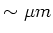from the closed orbit) will perform betatron oscillations around it; this motion is however damped by synchrotron radiation. Because the electrons do not necessarily pass quadrupole or sextupole magnets in their center, they see perpendicular fields in addition to the main bending field. Furthermore the electrons see the time-varying fields of kicker and corrector magnets as well as possible errors like misalignment of magnets or dipole rolls (i.e. when a dipole magnet's misalignment is a rotation around the design orbit). All these additional field components have to be considered in equation 11.

Longitudinal magnetic fields (found in insertion devices and in case of dipole pitch, i.e. when a dipole is aligned out of the horizontal plane) lead to Larmor precession of the spin around the electron's momentum axis resulting in a change of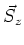. Radial magnetic fields (found in quadrupoles, sextupoles and vertical kicker magnets as well as in case of dipole rolls) lead to Thomas precession perpendicular to the electron's momentum axis, which again results in a change of(see figure 2).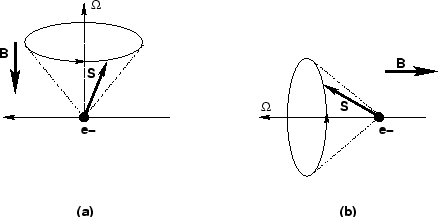Figure 2a: Thomas Precession. Figure 2b: Larmor Precession

If these additional fields in conjunction with spin diffusion result in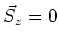we refer to a depolarized beam which has a polarization degree of zero3. The important difference between depolarizing effects is their energy dependency. As shown in equation 11 precession due to longitudinal fields depend inversely on the energy whereas radial and vertical fields lead to energy independent precession; therefore in high energy machines the longitudinal field effects can be neglected when compared to transverse fields.4

In order to depolarize the beam at SLS (flat machine) radial fields are needed. Applying the field from a time-varying vertical kicker magnet, the spin's precession cone angle is enlarged from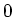towards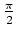. Together with the effect of spin diffusion this leads to the point wherewhen integrating over many electrons therefore resulting in zero polarization (see figure 3).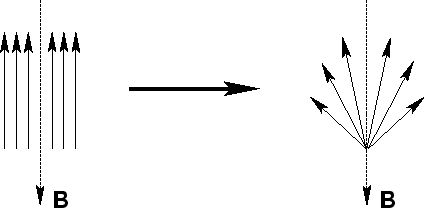Figure 3: The Spin diffusion process.

Assume a vertical kicker magnet applying to the electrons in the storage ring a radial magnetic field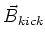. The precession frequency along the electron's momentum direction according to equations 11, 12 is given by: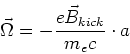(15)

This process is energy independent as already mentioned. But this kicker magnet's time varying magnetic field not only has an influence on the electron's spin, but also on the trajectory of the electrons. When passing the kicker magnet the electrons are kicked'' vertically from their trajectory and a betatron oscillation around the closed orbit is excited. Normally the betatron frequency is far enough away from the chosen kicker frequency, so no resonance conditions are fulfilled; therefore the beam's deviation from the closed orbit remains very small. Nevertheless the electrons will continue on their trajectory around the ring and pass a quadrupole magnet where their displacement from the closed orbit can lead to a modification of the depolarizing effects induced by the kicker magnet.

While passing the kicker magnet the electron obeys to the simple equation (see equation 12)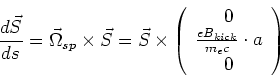(16)

which has the solution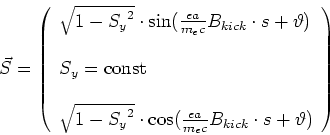(17)

When the electron passes the depolarizing kicker its spin precession cone angle is widened by: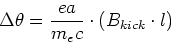(18)

where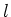is the length of the kicker. The kicker magnet actually kicks according to a sinusoidal waveform so we have to take in account that the real kicker strength is a time averaged value: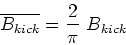(19)

and therefore the actual widening in the kicker (per revolution) is: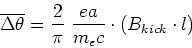(20)Next: Resonant Depolarization Up: Introduction Previous: Spin Dynamics   Contents
Simon Leemann
2002-03-15